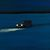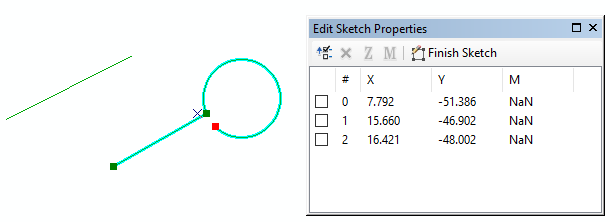# Determine if line has true curves via SQL (SDE.ST_GEOMETRY in Oracle)

405
1
02-03-2022 06:24 AM
Status: Openby
Frequent Contributor II

Currently, it's not possible to determine if a SDE.ST_GEOMETRY line has true curves via SQL.

For example, select lines where the SHAPE contains at least one true curve:Could ESRI consider adding a function to SDE.ST_GEOMETRY (Oracle) for this purpose?

Something like:

• SDE.ST_HasCurves()

Thanks.

1 Commentby

In the meantime, I wonder if this would be a possible workaround:

1. Create a field in the feature class called HAS_CURVES.

2. Populate the field in real-time/when a user creates or modifies a feature -- using a Calculation Attribute Rule.

• Compare \$feature.shape_length, which is the true length, including true curves
vs.
Length(\$feature), which is the pre-densified length, where true curves have been converted into straight segments.
https://i.stack.imgur.com/ilJrg.png

• If there is a difference between those two values, then that tells us that the line has curves. Use a Calculation Attribute Rule to set the HAS_CURVES field to "Y".

3. That field would be available to SQL. So we could query on it as needed.

Disclaimer: It's possible that comparing the those lengths might not work in all scenarios (due to rounding complications, etc.). Testing would be needed. As the idea suggests, it would be a lot more robust to have a SDE.ST_HasCurves() function in ST_GEOMETRY.

Related: